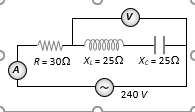Electrical Resonance series circuit
Question

# In the circuit shown in figure neglecting source resistance the voltmeter and ammeter reading will respectively, beModerate
Solution

## The voltage ${V}_{L}$ and ${V}_{C}$ are equal and opposite so voltmeter reading will be zero.Also$R=30\mathrm{\Omega },{X}_{L}={X}_{C}=25\mathrm{\Omega }$so $\mathrm{i}=\frac{\mathrm{V}}{\sqrt{{\mathrm{R}}^{2}+{\left({\mathrm{X}}_{\mathrm{L}}-{\mathrm{X}}_{\mathrm{C}}\right)}^{2}}}=\frac{\mathrm{V}}{\mathrm{R}}=\frac{240}{30}=8A$

Get Instant Solutions